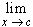#Interactive Real Analysis

Next | Previous | Glossary | Map

## 6.2. Continuous Functions

### Proposition 6.2.3: Continuity preserves Limits

If f is continuous at a point c in the domain D, and {xn} is a sequence of points in D converging to c, thenf(x) = f(c).

Iff(x) = f(c) for every sequence {xn} of points in D converging to c, then f is continuous at the point c.

### Proof:

The proof is very similar to the previous result about the equivalence of the two definitions of limits for a function. It is therefore left as an exercise. It would be good practice to see if you can modify the previous proof and adapt it to this result.

Next | Previous | Glossary | Map